Courses

# Test: Quadratic Equations - 3

## 25 Questions MCQ Test Mathematics (Maths) Class 10 | Test: Quadratic Equations - 3

Description
This mock test of Test: Quadratic Equations - 3 for Class 10 helps you for every Class 10 entrance exam. This contains 25 Multiple Choice Questions for Class 10 Test: Quadratic Equations - 3 (mcq) to study with solutions a complete question bank. The solved questions answers in this Test: Quadratic Equations - 3 quiz give you a good mix of easy questions and tough questions. Class 10 students definitely take this Test: Quadratic Equations - 3 exercise for a better result in the exam. You can find other Test: Quadratic Equations - 3 extra questions, long questions & short questions for Class 10 on EduRev as well by searching above.
QUESTION: 1

### Which of the following quadratic expression can be expressed as a product of real linear factors?

Solution: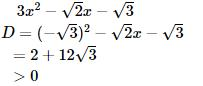Thus, it can be expressed as product of linear factors.

QUESTION: 2

Solution:
QUESTION: 3

### Solve for x : 15x2 – 7x – 36 = 0

Solution: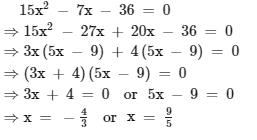QUESTION: 4

Solve for y : (√7) y2 – 6y –13 (√7) = 0

Solution: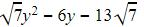Using mid term splitting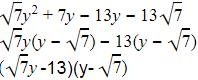So either y=13/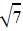Or y=QUESTION: 5

Solve for x : 6x2 + 40 = 31x

Solution:
QUESTION: 6

Determine k such that the quadratic equation x2 + 7(3 + 2k) – 2x (1 + 3k) = 0 has equal roots :

Solution:
QUESTION: 7

Discriminant of the equation – 3x2 + 2x – 8 = 0 is

Solution: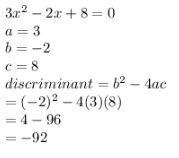QUESTION: 8

The nature of the roots of the equation x2 – 5x + 7 = 0 is –

Solution:

Given equation is x2-5x+7=0
We have discriminant as b2-4ac=(-5)2-4*1*7= -3
And x =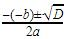, Since we do not have any real number which is a root of a negative number, the roots are not real.

QUESTION: 9

The roots of a2x2 + abx = b2, a = 0 are :

Solution:
QUESTION: 10

The equation x2 – px + q = 0 p, q ε R has no real roots if :

Solution:
QUESTION: 11

Determine the value of k for which the quadratic equation 4x2 – 3kx + 1 = 0 has equal roots :

Solution:
QUESTION: 12

Find the value of k such that the sum of the squares of the roots of the quadratic equation x2 – 8x + k = 0 is 40:

Solution:
QUESTION: 13

Find the value of p for which the quadratic equation x2 + p(4x + p – 1) + 2 = 0 has equal roots :

Solution:
QUESTION: 14

The length of a hypotenuse of a right triangle exceeds the length of its base by 2 cm and exceeds twice the length of the altitude by 1 cm. Find the length of each side of the triangle (in cm) :

Solution:
QUESTION: 15

A two digit number is such that the product of it's digits is 12. When 9 is added to the number, the digits interchange their places, find the number :

Solution:
QUESTION: 16

A plane left 40 minutes late due to bad weather and in order to reach it's destination, 1600 km away in time,it had to increase it's speed by 400 km/h from it's usual speed. Find the usual speed of the plane :

Solution:
QUESTION: 17

The sum of the squares of two consecutive positive odd numbers is 290. Find the sum of the numbers :

Solution:
QUESTION: 18

A shopkeeper buys a number of books for Rs. 80. If he had bought 4 more for the same amount, each book would have cost Re. 1 less. How many books did he buy?

Solution:
QUESTION: 19

Two squares have sides x cm and (x + 4) cm. The sum of their areas is 656 cm2. Find the sides of the square.

Solution:
QUESTION: 20

The real values of a for which the quadratic equation 2x2 – (a3 + 8a – 1) x + a2 – 4a = 0 possesses roots of opposite signs are given by :

Solution:
QUESTION: 21

The number of real solutions of the equation is :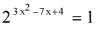Solution:
QUESTION: 22

If the equation (3x)2 + (27 × 31/k  – 15) x + 4 = 0 has equal roots, then k =

Solution:
QUESTION: 23

If x =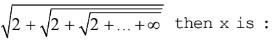Solution:
QUESTION: 24

Equation ax2 + 2x + 1 has one double root if :

Solution:
QUESTION: 25

Solve for x : (x + 2) (x – 5) (x – 6) (x + 1) = 144 :

Solution: